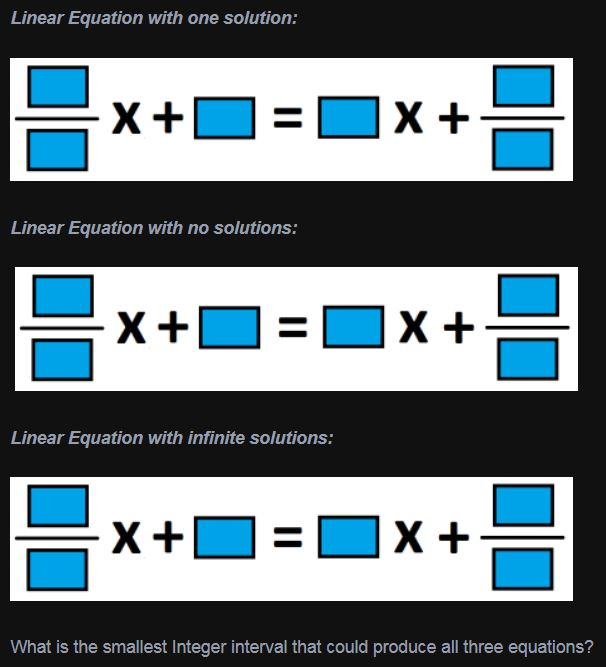# One Solution, No Solutions, Infinite Solutions

Directions: Using Integers (without repeating any number), fill in the boxes to create the following types of Linear Equations### Hint

What does it mean for an equation to have no solutions? Infinite solutions?

Answers can vary, an example is:
1 Solution: (-3/-1)X + 0 = 1X + (-4/-2)
No Solution: (-2/-1)X + 1 = 2X + 0
Infinite Solutions: (-2/-1)X + 3 = 2X + (-9/-3)

Interval of: 11

Source: Bryan Anderson

## Square Root Expression 2

Directions: Using the digits 0 to 9 at most one time each, place a digit …

1.Cannot make an entry. But I understand the three choices.

2.I think the smallest integer interval is 12. The numbers are from -9 to 3. 3-(-9)=12.

3.could not fill in the boxes but i know if slope is same and y is not there is no solution if y is same but slope is different 1 solution and all is the same means infinite solutions

4.i could not fill in the boxes but other then that i personally know that cant have if the slope is same and there is nothing for the y there is no solution but if alll are the same there are much more solutions

5.One solution
6/2 x + 5 = 2x + 8/1

No solution
6/2 x + 4 = 3x + 5/1

Infinite solution
10/2 x + 3 = 5x + 12/4

•No solution
6/2 x + 4 = 3x + 5/1

Infinite solution

One solution
6/2 x + 5 = 2x + 8/1
Infinite solution
10/2 x + 3 = 5x + 12/4

6.No solution
6/2 x + 4 = 3x + 5/1

Infinite solution
10/2 x + 3 = 5x + 12/4

One solution
6/2 x + 5 = 2x + 8/1